# Texas Instruments - Texas Instruments TI-Nspire CX II

Graphing calculator - USB - battery

•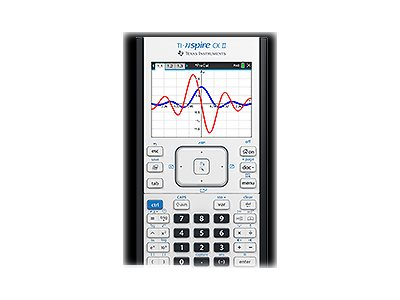•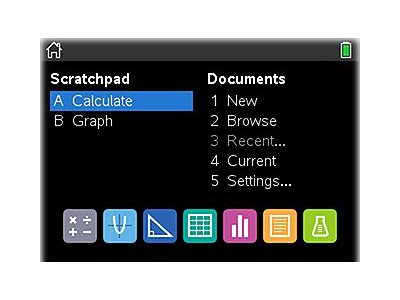•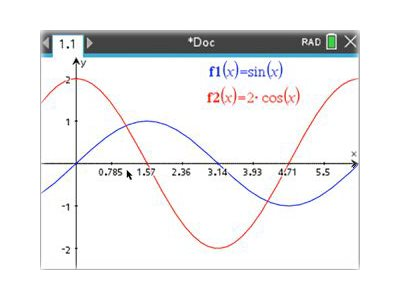•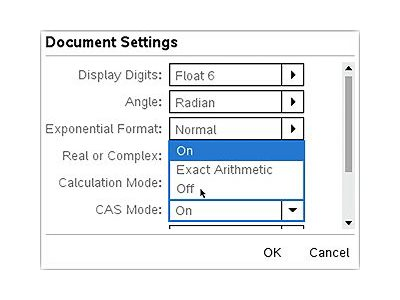•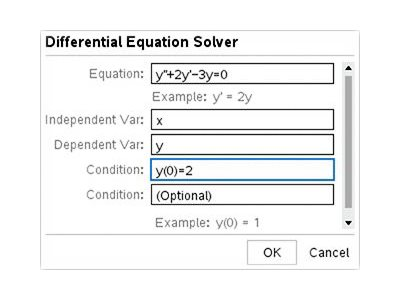•Texas Instruments
TINSPIRECXII
0033317208470
N/A
New

MSRP: \$236.45
In Stock

## Specifications

Main Specifications
Product Description Texas Instruments TI-Nspire CX II - graphing calculator
Product Type Graphing calculator - LCD
Interface USB
Basic Functions Addition, subtraction, multiplication, division, save, edit
Scientific Functions Binary, complex numbers, statistical analysis, linear regression, hypothesis testing, confidence intervals, matrix, logarithmic regression, exponential regression, power regression, differential, derivatives, inverse matrix, quadratic polynomial regression, cubic polynomial regression, quartic polynomial regression, median-median regression, logistic regression, sinusoidal regression, analysis of variance, determinant, transpose, chemical notation, symbolic units, normal distribution, inverse normal distribution, binomial distribution, poisson distribution
Graphing Functions 3D graphing, polar, sequence, zoom, parametric plot, histogram, scatter plot, plot statistics, box and whisker plot, geometric figure graphing
Diagonal Size 3.2"
Color Support Color
Power Source Battery
Compliant Standards FCC Part 15
General
Product Type Graphing calculator
Interface USB
Power Source Battery
RAM 64 MB
Flash Memory 90 MB
Miscellaneous
Included Accessories USB cable
Compliant Standards FCC Part 15
Warranty 1 year
Software
Software Included Notes, Calculator, Geometry, Lists & Spreadsheet, Data & Statistics, TI-Nspire CX Student Software, TI-Nspire CX Premium Teacher Software, TI-Nspire CX Navigator System
Display
Type LCD
Diagonal Size 3.2"
Color Support Color
Display Resolution 320 x 240 pixels
Dimensions & Weight
Width 3.4 in
Depth 7.5 in
Height 0.6 in
Weight 8.82 oz
Dimensions & Weight (Shipping)
Shipping Width 7.6 in
Shipping Depth 10.7 in
Shipping Height 2 in
Shipping Weight 18.34 oz
Functions
Basic Addition, subtraction, multiplication, division, save, edit
Scientific Binary, complex numbers, statistical analysis, linear regression, hypothesis testing, confidence intervals, matrix, logarithmic regression, exponential regression, power regression, differential, derivatives, inverse matrix, quadratic polynomial regression, cubic polynomial regression, quartic polynomial regression, median-median regression, logistic regression, sinusoidal regression, analysis of variance, determinant, transpose, chemical notation, symbolic units, normal distribution, inverse normal distribution, binomial distribution, poisson distribution
Graphing 3D graphing, polar, sequence, zoom, parametric plot, histogram, scatter plot, plot statistics, box and whisker plot, geometric figure graphing

## Related Products

Below are the optional products related to the selected product.